# Building wheel files in github actions

At work we are using a new databricks environment (claims based pop health related models). Databricks is very nice as a data querying environment, but it is challenging building well vetted code libraries in python. See the blog post Please don’t make me use databricks notebooks for an overview of the issues. (Other environments that make you write in notebooks, such as Apache Zeppelin, have pretty much all the same limitations.)

So we are still working out the design pattern for how to best write well vetted code. It is looking a bit like this workflow by menziess, I have been able to get dbconnect (and databricks-sql), installed on local windows machines. From there I can do all the usual junk – linting pre-commits, writing unit tests, etc. on my local machine. Then I push, and can do some final checks (or run a real life pipeline), in the databricks GUI environment.

One difference though is instead of doing Azure pipelines to build the wheel files, I am using Github Actions. To share I use my retenmod package as an example. The github action is pretty straightforward, and uses the same trick to push inside the action as I wrote about previously.

So here is the action code in-situ, but I can copy-paste the workflow right here in the blog to illustrate the yaml:

``````# Github actions to build
# and push wheel files
on:
push:
branches:
- main
- master

jobs:
build_wheel:
runs-on: ubuntu-latest
steps:
- uses: actions/checkout@v2
- name: Set up Python
uses: actions/setup-python@v2
with:
python-version: 3.9
- name: Build wheel and install
run: |
python -m pip install --user --upgrade build
python -m build
#pip install .
find ./dist/*.whl | xargs pip install
python simple_test.py
- name: Configure Git
run: |
git config --global user.email "apwheele@gmail.com"
git config --global user.name "apwheele"
- name: Commit and push wheel
run: |
git commit -m 'pushing new wheel'
git push``````

And then in your databricks notebooks, you can then have a locally scoped environment, so can have:

``%pip install ./dist/libname.whl``

At the front of your notebook. And then in a code cell, can then do:

``````import retenmod as rm
# do whatever rm functions from the library``````

Just like any normal python package. There are a few potential gotchas here. 1) I will need to write a python script to also edit `libname.whl` in the data pipelines whenever I update versions (my unix grep/sed fu is not up to task to grep out whl files). But that should be as simple as calling `python edit_files.py` inside the github action, and then amending the `git add .` to scoop up the edited files.

A second part is that with work repos, pushing inside the action is a bit trickier, so we need to work with personal access tokens/actions secrets and set the remote url for the push. It is tough for me to illustrate that with public repos though, so will have to wait until another blog post.

# Fitting a plateau effects model in scipy

Dealing with a few models recently that people fit non-linear effects (either via polynomials or splines), and the results are just on their face too curvy.

There is also a common social science trope where people fit a polynomial to some data, and that clearly exploratory model fitting exercise becomes a main focal point of the paper.

But there is one scenario I commonly see though for curves that I think makes sense for quite a bit of social science data – a plateau effect. See for example this Hipp article that finds a plateau effect for poverty -> crime rates. John though uses a cubic function later to fit these effects, so it curves back down – I think a more reasonable model would enforce monotonic constraints so it doesn’t dip back down in the tails of the data. (The same issue often happens with quadratic polynomials as well.) I have some other blog posts on segmented models as well that are subject to the same not being monotonic where they should be.

A plateau model is difficult to fit out of the box though in most current stat software. Rick Wicklin on his blog has a nice formulation though: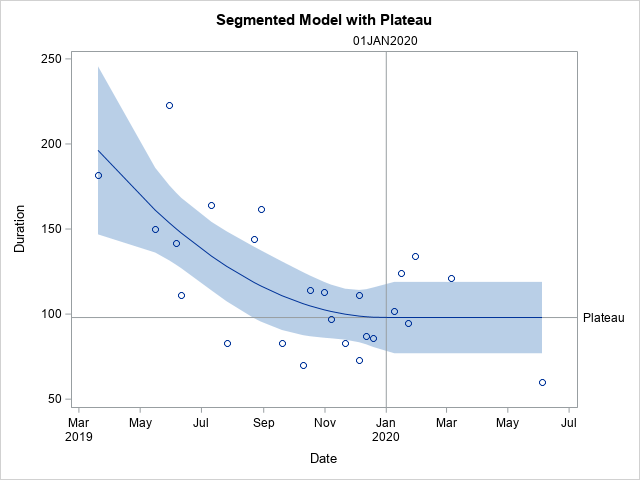It fits a quadratic, and then plateaus after a particular breakpoint. For theory testing I imagine the breakpoint itself will be of interest to many criminologists, and you can estimate that location in this formulation.

Rick works for SAS, and so if familiar with SAS go ahead and use his code. But here I coded up an example fitting a constrained non-linear regression in python using scipy.

# Python Code

Taking the same data from Rick Wicklin’s blog post, this code just reads in the data and converts dates to days since 3/20/2019. I don’t scale the data here to be an exact replicate of Rick’s blog post, but for data with a wider range it would be necessary to prevent some numerical instability.

``````# Python libraries to replicate

from datetime import datetime
import numpy as np
import pandas as pd
from scipy.optimize import minimize
from scipy.optimize import NonlinearConstraint

# Via https://blogs.sas.com/content/iml/2020/12/14/segmented-regression-sas.html
dat = [(1,'3/20/2019',182),
(3,'5/30/2019',223),
(5,'6/11/2019',111),
(7,'7/26/2019',83),
(9,'8/29/2019',162),
(11,'10/10/2019',70),
(13,'10/31/2019',113),
(15,'11/21/2019',83),
(17,'12/5/2019',73),
(19,'12/19/2019',86),
(21,'1/16/2020',124),
(23,'1/30/2020',134),
(25,'6/4/2020',60),
(2,'5/16/2019',150),
(4,'6/6/2019',142),
(6,'7/11/2019',164),
(8,'8/22/2019',144),
(10,'9/19/2019',83),
(12,'10/17/2019',114),
(14,'11/7/2019',97),
(16,'12/5/2019',111),
(18,'12/12/2019',87),
(20,'1/9/2020',102),
(22,'1/23/2020',95),
(24,'3/5/2020',121)]

df = pd.DataFrame(dat,columns=['SugeryNo','Date','Duration'])
df['Date'] = pd.to_datetime(df['Date'])
df['DaysRef'] = (df['Date'] - pd.to_datetime('3/20/2019')).dt.days
df['DR2'] = df['DaysRef']**2``````

Now, one of the things I sometimes find confusing in posts that optimize arbitrary functions (in R or python) is that to minimize a function, it is with respect to your data at hand. Sometimes folks have functions that can pass in data and the parameters. But I find it easier to just keep the data fixed and only pass in parameters.

So you can see in my non-linear pred function, it passes in the parameters (which we will estimate), and gives a prediction for the fixed dataset. Ditto for the loss function (you could update to do logistic regression for example if predicting 0/1s). Then the `nlconst` object is a special python function to define the non-linear constraints that make this plateau model work. Then start solutions and finally minimize the function (using a Fortran solver!):

``````# Pass in global data into the function
def prednl(x):
b0 = x
b1 = x
b2 = x
brp = x
before = (df['DaysRef'] < brp)
y0 = b0 + b1*df['DaysRef'] + b2*df['DR2']
y1 = b0 + b1*brp + b2*brp*brp
return y0*before + (~before)*y1

def lossnl(x):
yhat = prednl(x)
squares = (df['Duration'] - yhat)**2
return squares.sum()

def nlconst(x):
r1 = x - (x + x*x + x*x*x)    # plateau
r2 = x - ((-0.5*x)/x)                     # breakpoint
# Could also consider bounds on breakpoint and curve needs to be non-zero
return np.array([r1,r2])

nlc = NonlinearConstraint(nlconst, np.array([0.0,0.0]),
np.array([0.0,0.0]))

start = np.array([185.0,-1.0,0.1,150.0,60.0])

solution = minimize(lossnl,start,method='trust-constr',
constraints=nlc,options={'maxiter':50000})``````

And this returns the same fit as did the SAS routine: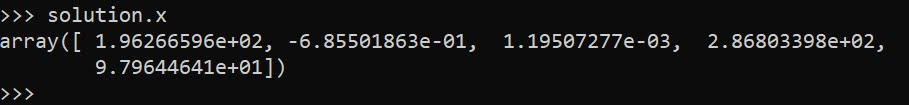Now I will admit defeat to trying to figure out analytical standard errors (tried via the outer product gradient approach via `autograd`, as well as using BFGS and its inverse hessian estimate, which is not even close to the results SAS gives).

So I do the thing all lazy statisticians do at this point – the bootstrap. (SPSS I believe will only give standard errors for its nonlinear estimates via bootstrap.)

``````# Do the bootstrap, 95% CI
res = []
mess = []
for i in range(19):
print(f'iter {i+1}: ',datetime.now())
boot = df.sample(n=df.shape,replace=True).reset_index(drop=True)
days_ref = boot['DaysRef'].to_numpy()
duration = boot['Duration'].to_numpy()
dr2 = boot['DR2'].to_numpy()
def lb(x):
b0 = x
b1 = x
b2 = x
brp = x
before = (days_ref < brp)
y0 = b0 + b1*days_ref + b2*dr2
y1 = b0 + b1*brp + b2*brp*brp
yhat = y0*before + (~before)*y1
squares = (duration - yhat)**2
return squares.sum()
sl = minimize(lb,start,method='trust-constr',
constraints=nlc,options={'maxiter':50000})
mess.append(sl.message)
print(sl.message)
res.append(sl.x)

rdf = pd.DataFrame(res,columns=['B0','B1','B2','break','plateau'])
rdf.describe() #min/max are the 95% CIs``````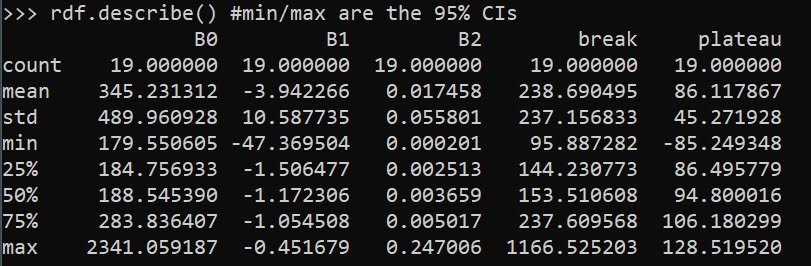And we can see that these estimates are very wide. We can look at individual iterations, and in a few the estimates go off the rails (and they still say they converged, they just converged to non-sense).

``````# Some of the wayward estimates
# still pass convergence
rdf['Eval'] = mess
rdf``````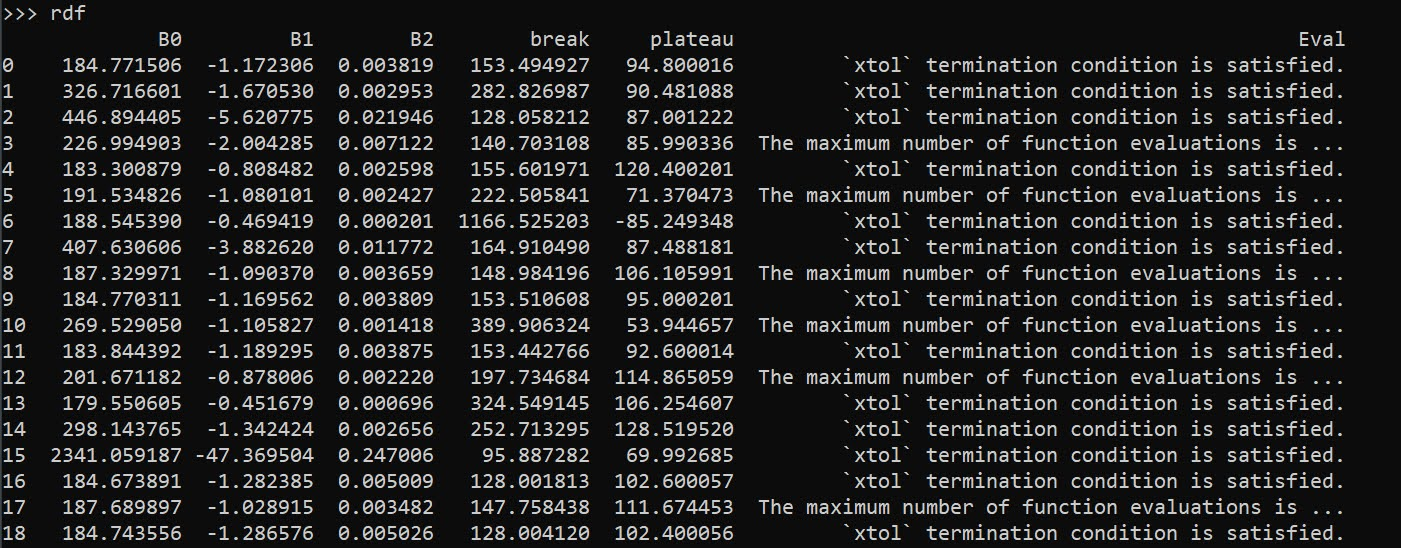But this is the nature of these non-linear functions. They can be pretty finicky. If a straight line fits the data quite well, the quadratic term will be very small, and so the estimated plateau may be outside of the data (or just totally unstable).

Still, even though it is more work and potentially more finicky in model fitting, I would rather people have explicit functional form predictions for non-linear effects, than simply throwing in polynomial functions and writing a paper about “look at these non-linear effects”.

And this formulation provides an explicit mechanism to measure the location of a plateau effect directly as a parameter.

# Some more github action tricks

Hackernews shared the other day a project using github actions to generate a nice readme for your base Github profile. That workflow uses rust to query the github API and get some stats to then insert into the README.

Two things I noticed I did not realize you could do with actions previously; 1) you can schedule actions to run on a regular basis via a cron job, 2) you can push to the repo inside of the action. (And this does not cause some infinite recursion with actions.) So I have updated my profile to run some python code, generating an image of the number of potholes filled in Raleigh per week.And you can see that this was updated on 4/7, and that was the automated job that was re-run.

It is pretty simple python code. You just have to have a step in your actions to build the python environment, then you can run your code.

With the regular cron job, you could offload different pieces of work to github, say automate scraping a site or sending out emails once a week. You just need to have a python (or whatever language) script to automate that process. Or you could do more fancy analysis for a project, and post that in the readme via a Jupyter notebook script. If the source data can be downloaded via the internet anyway.

So Gainwell has let me open source one of the projects I have been working on at work – a python package to download SVI data. The SVI is an index created by the CDC to identify areas of high health risk in four domains based on census data (from the American Community Survey).For my criminologist friends, these are very similar variables we typically use to measure social disorganization (see Wheeler et al., 2018 for one example criminology use case). It is a simple python install, `pip install svi-data`. And then you can get to work. Here is a simple example downloading zip code data for the entire US.

``````import numpy as np
import pandas as pd
import svi_data

key = svi_data.get_key('census_api.txt')

svi_zips = svi_data.get_svi(key,'zip',2019)
svi_zips['zipcode'] = svi_zips['GEO_ID'].str[-5:]``````

Note I deviate from the CDC definition in a few ways. One is that when I create the themes, instead of using percentile rankings, I z-score the variables instead. It will likely result in very similar correlations, but this is somewhat more generalizable across different samples. (I also change the denominator for single parent heads of households to number of families instead of number of households, I think that is likely just an error on CDC’s part.)

## Summed Index vs PCA

So here quick, lets check out my z-score approach versus a factor analytic approach via PCA. Here I just focus on the poverty theme:

``````pov_vars = ['EP_POV','EP_UNEMP','EP_PCI','EP_NOHSDP','RPL_THEME1']
svi_pov = svi_zips[['zipcode'] + pov_vars ].copy()

from sklearn import decomposition
from sklearn.preprocessing import scale

svi_pov.corr()``````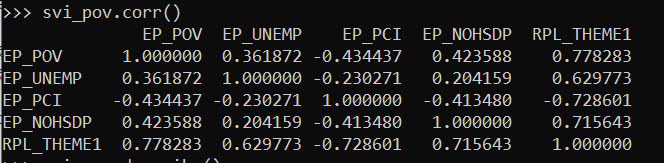Note the per capita income has a negative correlation, but you can see the index works as expected – lower correlations for each individual item, but fairly high correlation with the summed index.

Lets see what the index would look like if we used PCA instead:

``````pca = decomposition.PCA()
sd = scale(svi_pov[pov_vars[:-1]])
pc = pca.fit_transform(sd)
svi_pov['PC1'] = pc[:,0]
svi_pov.corr() #almost perfect correlation``````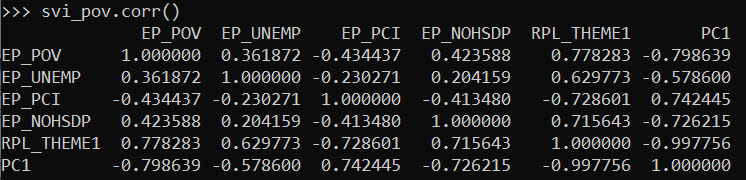You can see that besides the negative value, we have an almost perfect correlation between the first principal component vs the simpler sum score.

One benefit of PCA though is a bit more of a structured approach to understand the resulting indices. So we can see via the Eigen values that the first PC only explains about 50% of the variance.

``print(pca.explained_variance_ratio_)``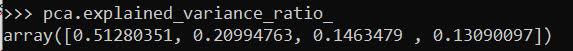``````comps = pca.components_.T
cols = ['PC' + str(i+1) for i in range(comps.shape)]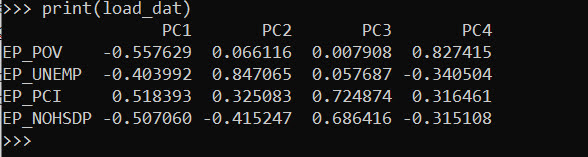So for PC3 for example, it has areas with high no highschool, as well as high per capita income. So higher level components can potentially identify more weird scenarios, which healthcare providers probably don’t care about so much by is a useful thing to know for exploratory data analysis.

## Mapping

Since these are via census geographies, we can of course map them. (Here I grab zipcodes, but the code can download counties or census tracts as well.)

We can download the census geo data directly into geopandas dataframe. Here I download the zip code tabulation areas, grab the outline of Raleigh, and then only plot zips that intersect with Raleigh.

``````import geopandas as gpd
import matplotlib.pyplot as plt

# Getting the spatial zipcode tabulation areas
zip_url = r'https://www2.census.gov/geo/tiger/TIGER2019/ZCTA5/tl_2019_us_zcta510.zip'
zip_geo.rename(columns={'GEOID10':'zipcode'},inplace=True)

# Merging in the SVI data
zg = zip_geo.merge(svi_pov,on='zipcode')

# Getting outline for Raleigh
ncp_url = r'https://www2.census.gov/geo/tiger/TIGER2019/PLACE/tl_2019_37_place.zip'
ral = ncp_geo[ncp_geo['NAME'] == 'Raleigh'].copy()
ral_proj = 'EPSG:2278'
ral_bord = ral.to_crs(ral_proj)

ral_zips = gpd.sjoin(zg,ral,how='left')
ral_zips = ral_zips[~ral_zips['index_right'].isna()].copy()
ral_zipprof = ral_zips.to_crs(ral_proj)

# Making a nice geopandas static map, zoomed into Raleigh

fig, ax = plt.subplots(figsize=(6,6), dpi=100)

# Raleighs boundary is crazy
#ral_bord.boundary.plot(color='k', linewidth=1, edgecolor='k', ax=ax, label='Raleigh')
ral_zipprof.plot(column='RPL_THEME1', cmap='PRGn',
legend=True,
edgecolor='grey',
ax=ax)

# via https://stackoverflow.com/a/42214156/604456
ral_zipprof.apply(lambda x: ax.annotate(text=x['zipcode'], xy=x.geometry.centroid.coords, ha='center'), axis=1)

ax.get_xaxis().set_ticks([])
ax.get_yaxis().set_ticks([])

plt.show()``````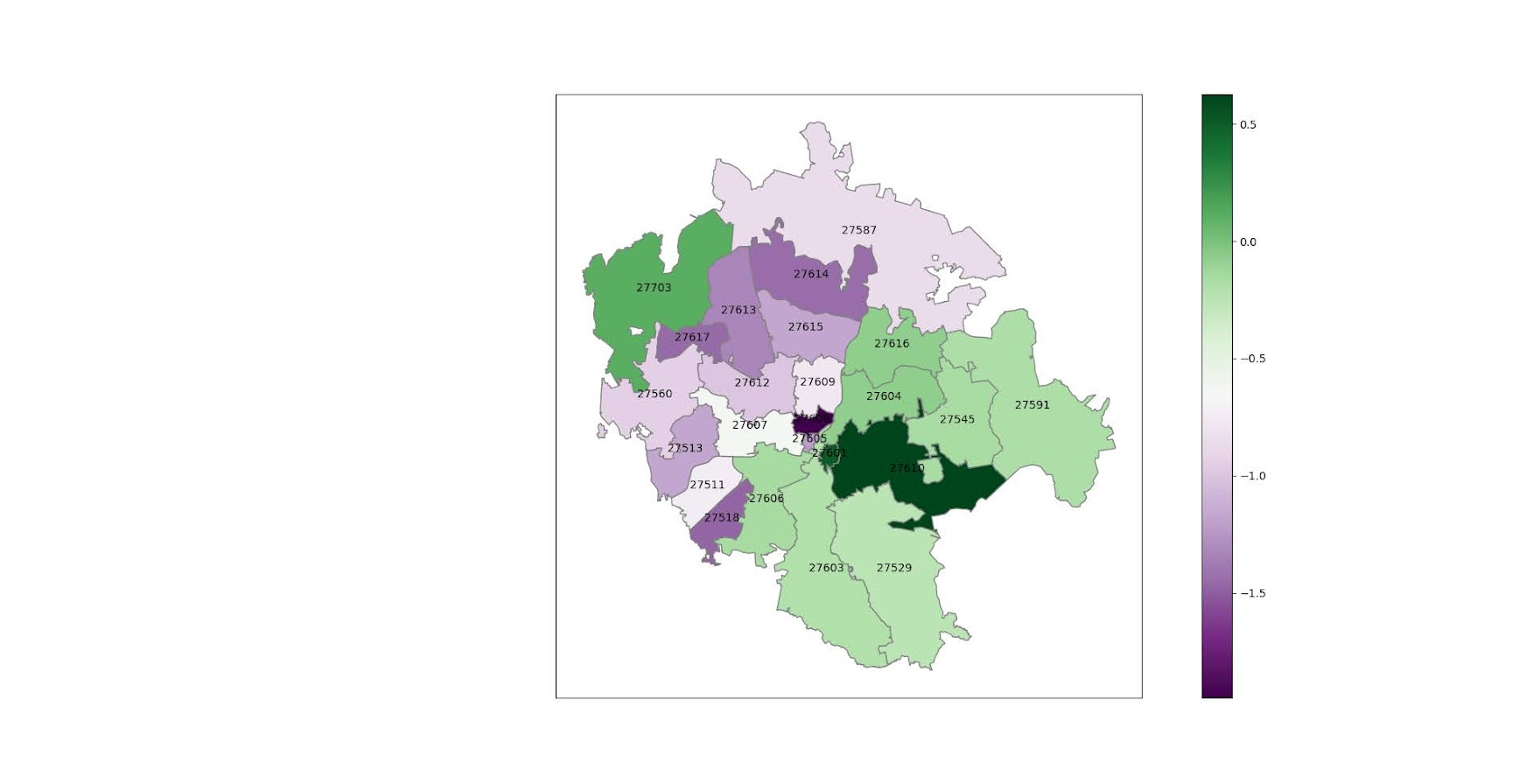I prefer to use smaller geographies when possible, so I think zipcodes are about the largest areas that are reasonable to use this for (although I do have the ability to download this for counties). Zipcodes since they don’t nicely overlap city boundaries can cause particular issues in data analysis as well (Grubesic, 2008).

# Other Stuff

I have a notebook in the github repo showing how to grab census tracts, as well as how to modify the exact variables you can download.

It does allow you to specify a year as well (in the notebook I show you can do the 2018 SVI for the 16/17/18/19 data at least). Offhand for these small geographies I would only expect small changes over time (see Miles et al., 2016 for an example looking at SES).

One of the things I think has more value added (and hopefully can get some time to do more on this at Gainwell), is to peg these metrics to actual health outcomes – so instead of making an index for SES, look at micro level demographics for health outcomes, and then post-stratify based on census data to get estimates across the US. But that being said, the SVI often does have reasonable correlations to actual geospatial health outcomes, see Learnihan et al. (2022) for one example that medication adherence the SVI is a better predictor than distance for pharmacy for example.

# Use circles instead of choropleth for MSAs

We are homeschooling the kiddo at the moment (the plunge was reading by Bryan Caplan’s approach, and seeing with online schooling just how poor middle school education was). Wife is going through AP biology at the moment, and we looked up various job info on biomedical careers. Subsequently came across this gem of a map of MSA estimates from the Bureau of Labor Stats (BLS) Occupational Employment and Wage Stats series (OES).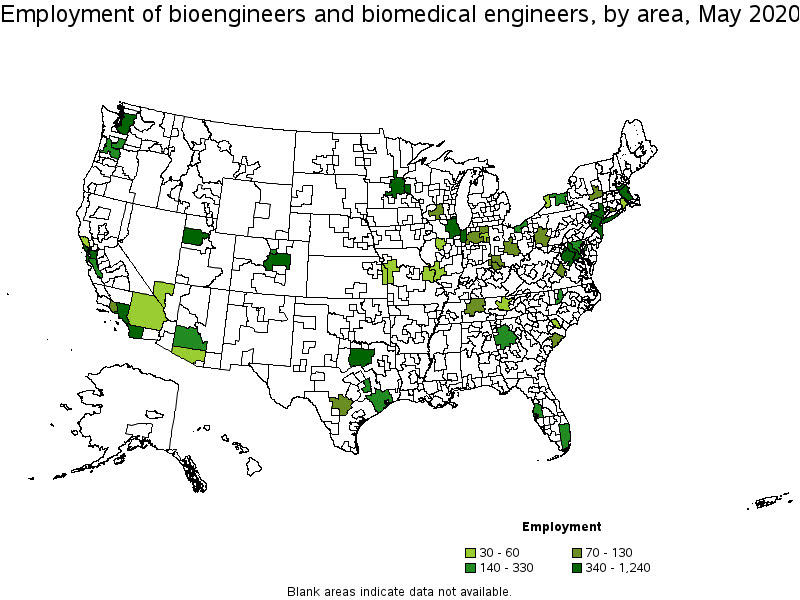I was actually mapping some metro stat areas (MSAs) at work the other day, and these are just terrifically bad geo areas to show via a choropleth map. All choropleth maps have the issue of varying size areas, but I never realized having somewhat regular borders (more straight lines) makes the state and county maps not so bad – these MSA areas though are tough to look at. (Wife says it scintillates for her if she looks too closely.)

There are various incredibly tiny MSAs next to giant ones that you will just never see in these maps (no matter what color scheme you use). Nevada confused for me quite a bit, until I zoomed in to see that there are 4 areas, Reno is just a tiny squib.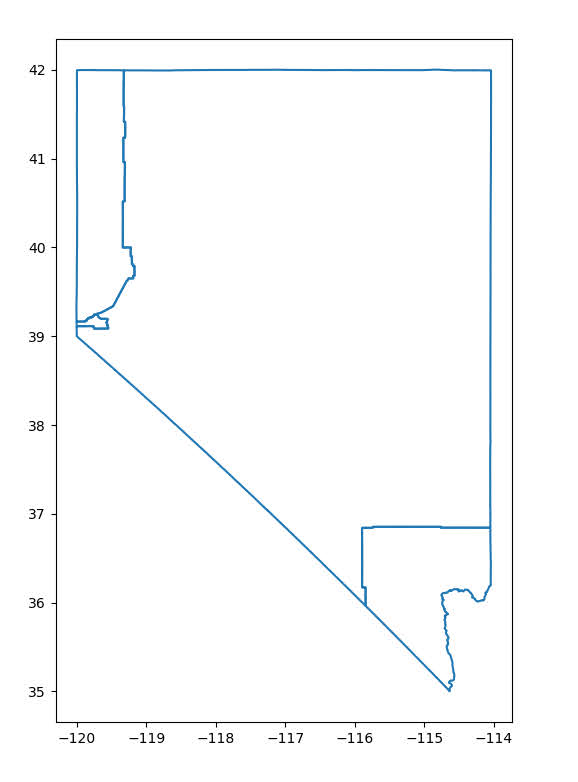Another example is Boulder above Denver. (Look closely at the BLS map I linked, you can just make out Boulder if you squint, but I cannot tell what color it corresponds to in the legend.) The outline heavy OES maps, which are mostly missing data, are just hopeless to display like this effectively. Reno could be the hottest market for whatever job, and it will always be lost in this map if you show employment via the choropleth approach. So of course I spent the weekend hacking together some maps in python and folium.

The BLS has a public API, but I was not able to find the OES stats in that. But if you go through the motions of querying the data and muck around in the source code for those queries, you can see they have an undocumented API call to generate json to fill the tables. Then using this tool to convert the json calls to python (thank you Hacker News), I was able to get those tables into python.

I have these functions saved on github, so check out that source for the nitty gritty. But just here quickly, here is a replicated choropleth map, showing the total employees for bio jobs (you can go to here to look up the codes, or run my function `bls_maps.ocodes()` to get a pandas dataframe of those fields).

``````# Creating example bls maps
from bls_geo import *

# can check out https://www.bls.gov/oes/current/oes_stru.htm
bio = '172031'
bio_stats = oes_geo(bio)
areas = get_areas() # this takes a few minutes
state = state_albers()
geo_bio = merge_occgeo(bio_stats,areas)

ax = geo_bio.plot(column='Employment',cmap='inferno',legend=True,zorder=2)
state.boundary.plot(ax=ax,color='grey',linewidth=0.5,zorder=1)
ax.set_ylim(0.1*1e6,3.3*1e6)
ax.set_xlim(-0.3*1e7,0.3*1e7)   # lower 48 focus (for Albers proj)
ax.set_axis_off()
plt.show()``````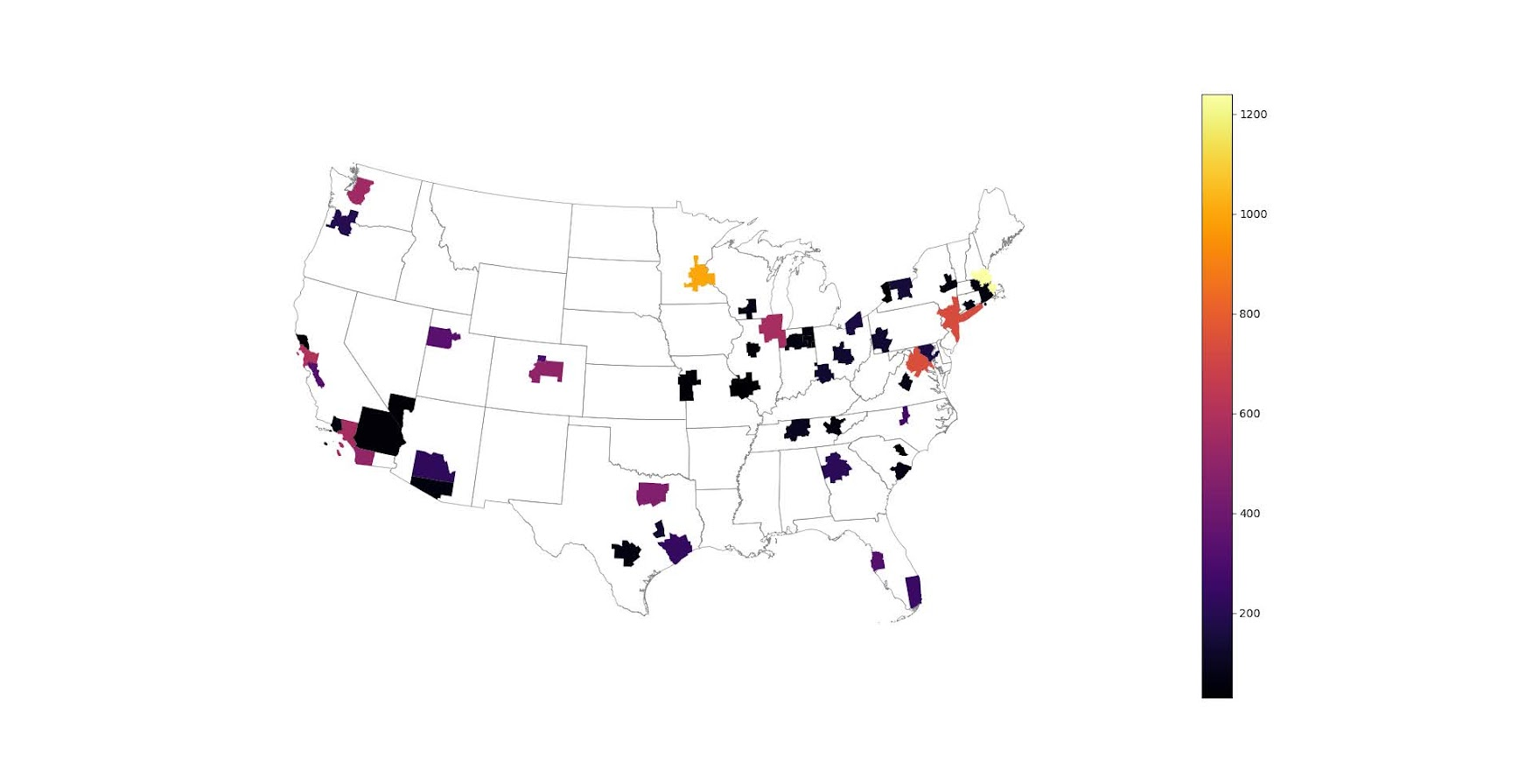And that is not much better than BLSs version. For this data, if you are just interested in looking up or seeing the top metro areas, just doing a table, e.g. above `geo_bio.to_excel('biojobs.xlsx')`, works just as well as a map.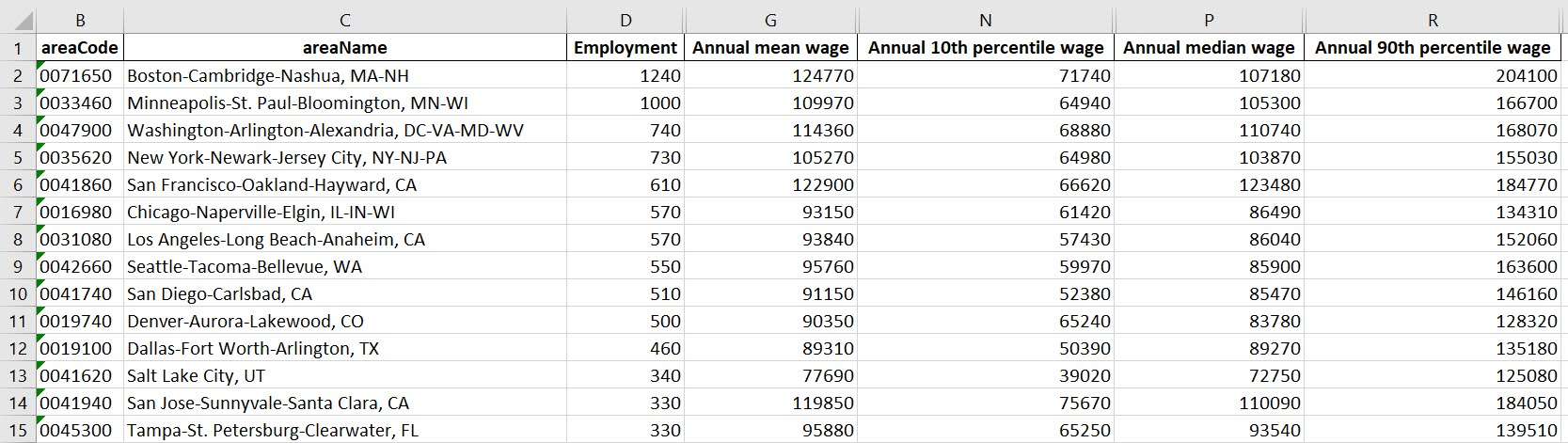So I was surprised to see Minneapolis pop up at the top of that list (and also surprised Raleigh doesn’t make the list at all, but Durham has a few jobs). But if you insist on seeing spatial trends, I prefer to go the approach of mapping proportion or graduate circles, placing the points at the centroid of the MSA:

``````att = ['areaName','Employment','Location Quotient','Employment per 1,000 jobs','Annual mean wage']
form = ['',',.0f','.2f','.2f',',.0f']

map_bio = fol_map(geo_bio,'Employment',['lat', 'lon'],att,form)
#map_bio.save('biomap.html')
map_bio #if in jupyter can render like this``````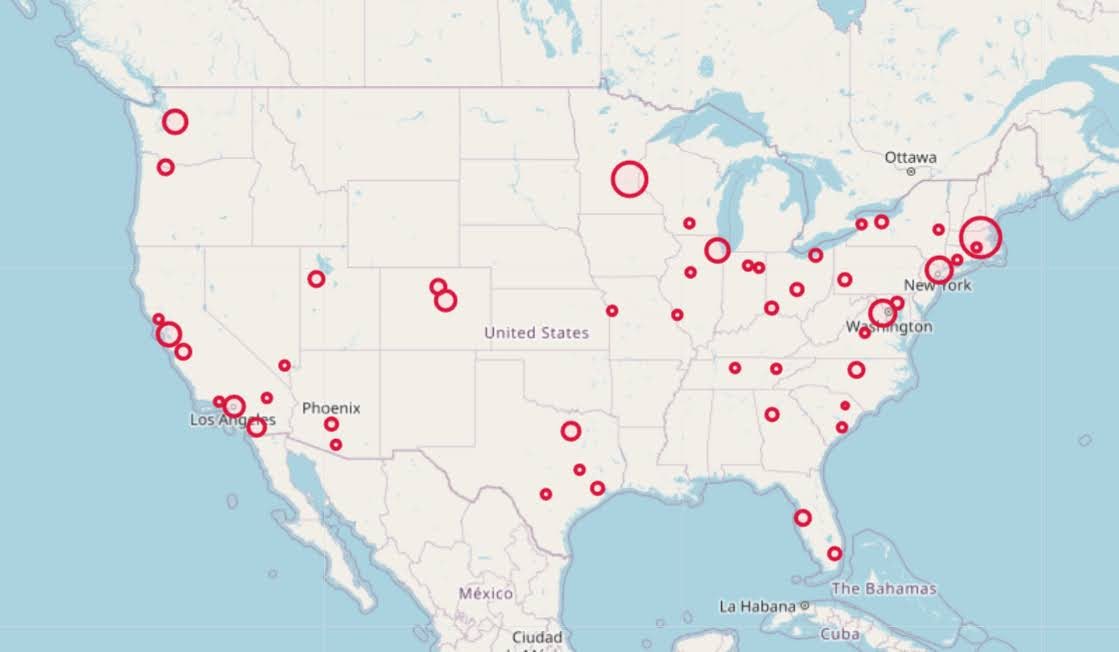I am too lazy to make a legend, you can check out nbviewer to see an interactive Folium map, which I have tool tips (similar to the hover for the BLS maps).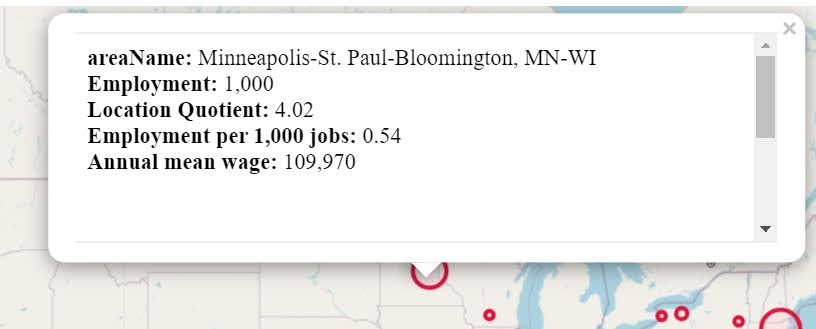Forgive my CSS/HTML skills, not sure how to make nicer popups. So you lose the exact areas these MSA cover in this approach, but I really only expect a general sense from these maps anyway.

These functions are general enough for whatever wage series you want (although these functions will likely break when the 2021 data comes out). So here is the OES table for data science jobs: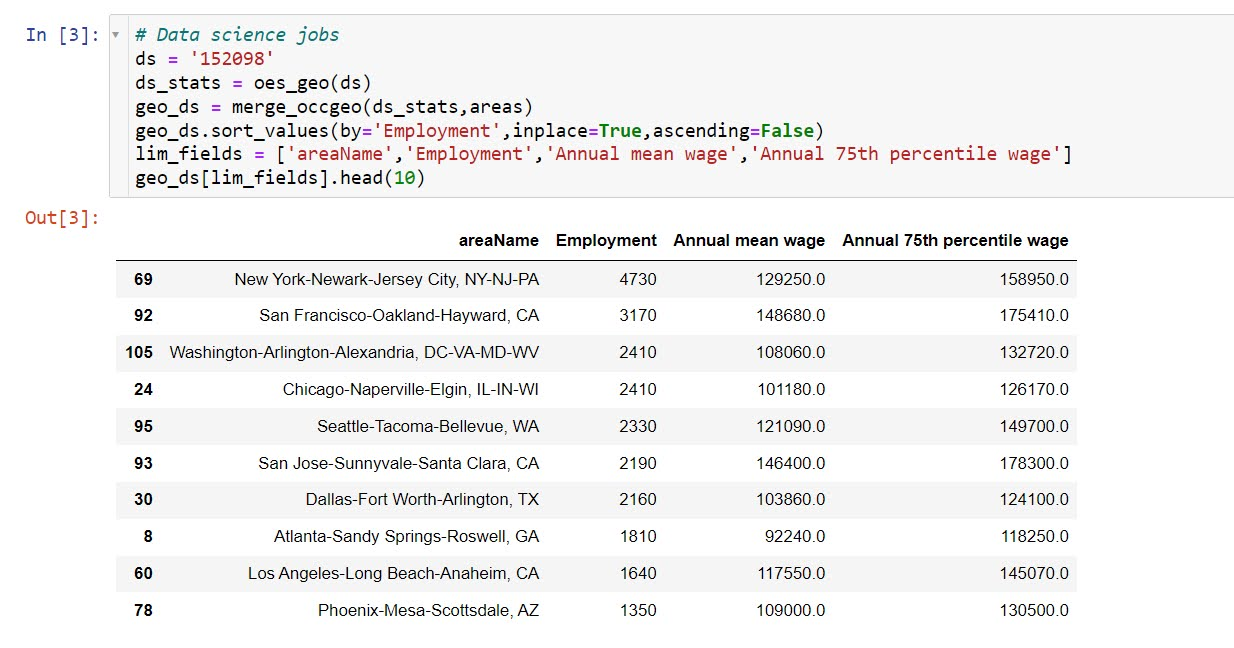I feel going for the 90th percentile (mapping that to the 10 times programmer) is a bit too over the top. But I can see myself reasonably justifying 75th percentile. (Unfortunately these agg tables don’t have a way to adjust for years of experience, if you know of a BLS micro product I could do that with let me know!). So you can see here the somewhat inflated salaries for the SanFran Bay area, but not as inflated as many might have you think (and to be clear, these are for 2020 survey estimates).

If we look at map of data science jobs, varying the circles by that 75th annual wage percentile, it looks quite uniform. What happens is we have some real low outliers (wages under 70k), resulting in tiny circles (such as Athen’s GA). Most of the other metro regions though are well over 100k.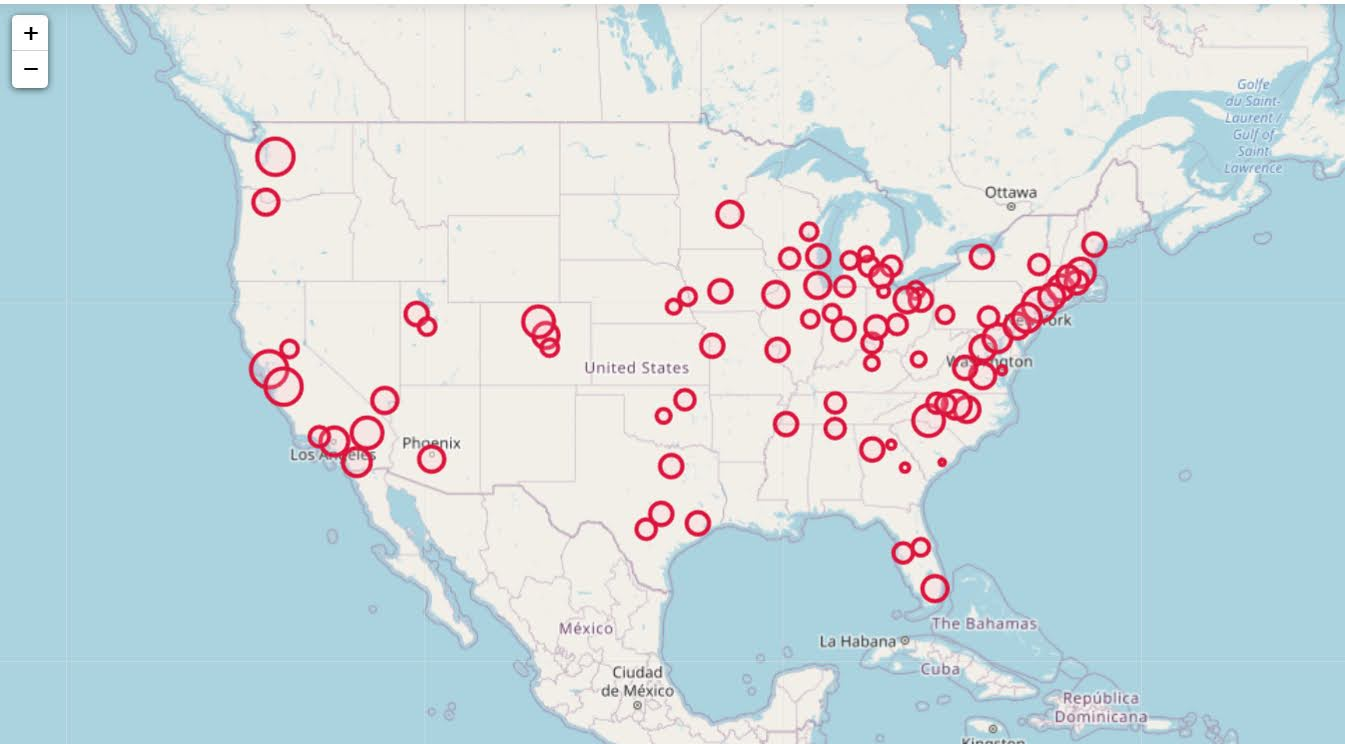In more somber news, those interactive maps are built using Leaflet as the backend, which was create by a Ukranian citizen, Vladimir Agafonkin. We can do amazing things with open source code, but we should always remember it is on the backs of someones labor we are able to do those things.

# Simulating data with numpy and scipy

I’ve answered a few different questions on forums recently about simulating data:

I figured it would be worth my time to put some of my notes down in a blog post. It is not just academic, not too infrequently I use simulations at work to generate estimated return on investment estimates for putting a machine learning model into production. For social scientists this is pretty much equivalent to doing cost/benefit policy simulations.

Simulations are also useful for testing the behavior of different statistical tests, see prior examples on my blog for mixture trajectories or random runs.

Here I will be showing how to mostly use the python libraries numpy and scipy to do various simulation tasks. For some upfront (note I set the seed in numpy, important for reproducing your simulations):

``````import itertools as it
import numpy as np
import pandas as pd
np.random.seed(10)

# total simulations for different examples
n = 10000

# helper function to pretty print values
def pu(x,ax=1):
te1,te2 = np.unique(x.sum(axis=ax),return_counts=True)
te3 = 100*te2/te2.sum()
res = pd.DataFrame(zip(te1,te2,te3),columns=['Unique','Count','Percent'])
return res``````

## Sampling from discrete choice sets

First, in the linked data science post, the individual had a very idiosyncratic set of simulations. Simulate a binary vector of length 10, that had 2,3,4 or 5 ones in that vector, e.g. `[1,1,0,0,0,0,0,0,0,0]` is a valid solution, but `[0,0,0,0,1,0,0,0,0,0]` is not, since the latter only has 1 `1`. One way to approach problems like these is to realize that the valid outcomes are a finite number of discrete solutions. Here I use itertools to generate all of the possible permutations (which can easily fit into memory, only 627). Then I sample from that set of 627 possibilities:

``````# Lets create the whole sets of possible permutation lists
res = []
zr = np.zeros(10)
for i in range(2,6):
for p in it.combinations(range(10),i):
on = zr.copy()
on[list(p)] = 1
res.append(on.copy())

resnp = np.stack(res,axis=0)

# Now lets sample 1000 from this list
total_perms = resnp.shape
samp = np.random.choice(total_perms,n)
res_samp = resnp[samp]

# Check to make sure this is OK
pu(res_samp)``````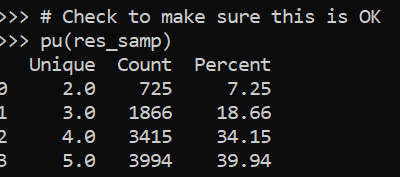Ultimately you want the simulation to represent reality. So pretend this scenario was we randomly pick a number out of the set {2,3,4,5}, and then randomly distribute the 1s in that length 10 vector. In that case, this sampling procedure does not reflect reality, because 2’s have fewer potential permutations than do 5’s. You can see this in the simulated proportions of rows with 2 (7.25%) vs rows with 5 (39.94%) in the above simulation.

We can fix that though by adjusting the sampling probabilities from the big set of 627 possibilities though. Pretty much all of the `np.random` methods an option to specify different marginal probabilities, where in choice it defaults to equal probability.

``````# If you want the different subsets to have equal proportions
sum_pop = resnp.sum(axis=1)
tot_pop = np.unique(sum_pop,return_counts=True)
equal_prop = 1/tot_pop.shape
pop_prob = pd.Series(equal_prop/tot_pop,index=tot_pop)
long_prob = pop_prob[sum_pop]

samp_equal = np.random.choice(total_perms,n,p=long_prob)
res_samp_equal = resnp[samp_equal]
pu(res_samp_equal)``````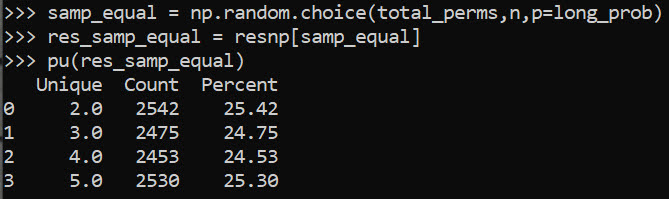So now we can see that each of those sets of results have similar marginal proportions in the simulation.

You can often figure out exact distributions for your simulations, for an example of similar discrete statistics, see my work on small sample Benford tests. But I often use simulations to check my math even if I do know how to figure out the exact distribution.

Another trick that I commonly use in other applications that don’t have access to something equivalent to `np.random.choice`, but most applications have a random uniform generator. Basically you can generate random numbers on whatever interval, chunk those up into bits, and turn those bits into your resulting categories. This is what I did in that SPSS post at the beginning.

``````unif = np.floor(np.random.uniform(1,33,(32*n,1)))/2
pu(unif)``````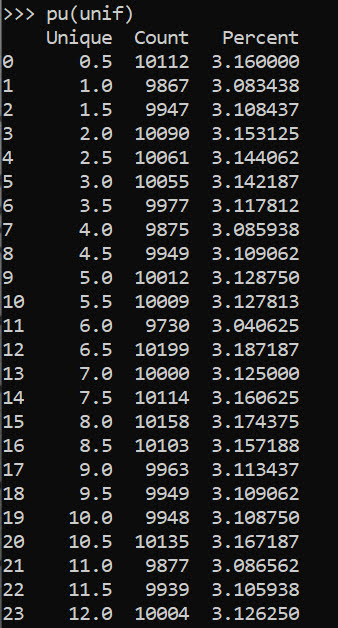But again this is not strictly necessary in python because we can generate the set/list of items and sample from that directly.

``````# Same as using random choice from that set of values
half_vals = np.arange(1,33)/2
unif2 = np.random.choice(half_vals,(32*n,1))
pu(unif2)``````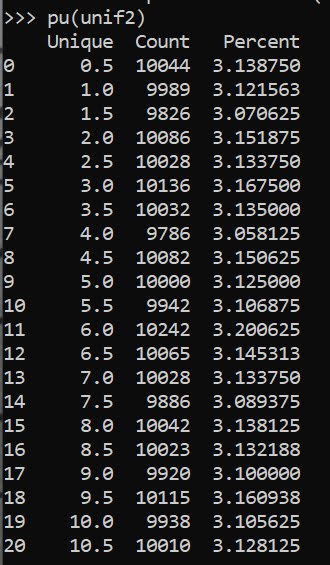But if limited in your libraries that is a good trick to know. Note that most random number generators operate over `(0,1)`, so open intervals and will never generate an exact 0 or 1. To get a continuous distribution over whatever range, you can just multiply the 0/1 random number generator (and subtract if you need negative values) to match the range you want. But again most programs let you input min/max in a uniform number generator.

## Sampling different stat distributions

So real data often can be reasonably approximated via continuous distributions. If you want to generate different continuous distribtions with approximate correlations, one approach is to:

• generate multi-variate standard normal data (mean 0, variance 1, and correlations between those variables)
• turn that matrix into a standard uniform via the CDF function
• then for each column, use the inverse CDF function for the distribution of choice

Here is an example generating a uniform 0/1 and a Poisson with a mean of 3 variable using this approach.

``````from scipy.stats import norm, poisson

# Here generate multivariate standard normal with correlation 0.2
# then transform both to uniform
# Then transform 2nd column to Poisson mean 3

mu = [0,0]
cv = [[1,0.2],[0.2,1]] #needs to be symmetric
mv = np.random.multivariate_normal([0,0],cov=cv,size=n)
umv = pd.DataFrame(norm.cdf(mv),columns=['Uniform','Poisson'])
umv['Poisson'] = poisson.ppf(umv['Poisson'],3)
print(umv.describe())``````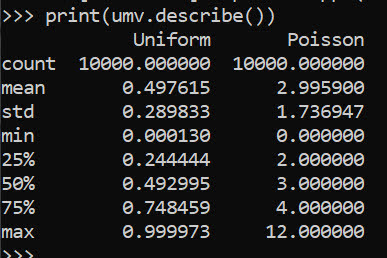This of course doesn’t guarantee the transformed data has the exact same correlation as the original specified multi-variate normal. (If interested in more complicated scenarios, it will probably make sense to check out copulas.)

Like I mentioned in the beginning, I am often dealing with processes of multiple continuous model predictions. E.g. one model that predicts a binary ‘this claim is bad’, and then a second model predicting ‘how bad is this claim in terms of \$\$\$’. So chaining together simulations of complicated data (which can be mixtures of different things) can be useful to see overall behavior of a hypothetical system.

Here I chain together a predicted probability for 3 claims (20%,50%,90%), and mean/standard deviations of (1000,100),(100,20),(50,3). So pretend we can choose 1 of these three claims to audit. We have the predicted probability that the claim is wrong, as well as an estimate of how much money we would make if the claim is wrong (how wrong is the dollar value).

The higher dollar values have higher variances, so do you pick the safe one, or do you pick the more risky audit with higher upside. We can do a simulation to see the overall distribution:

``````pred_probs = np.array([0.2,0.5,0.9])
bin_out = np.random.binomial(n=1,p=pred_probs,size=(n,3))
print( bin_out.mean(axis=0) )

# Pretend have both predicted prob
# and distribution of values
# can do a simulation of both

val_pred = np.array([1000,100,50])
val_std = np.array([100,20,3])
val_sim = np.random.normal(val_pred,val_std,size=(n,3))
print(val_sim.mean(axis=0))
print(val_sim.std(axis=0))

revenue = val_sim*bin_out
print(revenue.mean(axis=0))
print(revenue.std(axis=0))``````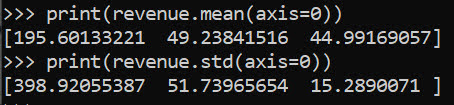I could see a reasonably risk averse person picking the lowest dollar claim here to audit. Like I said in the discrete case, we can often figure out exact distributions. Here the expected value is easy, `prob*val`, the standard deviation is alittle more tricky to calculate in your head (it is a mixture of a spike at 0 and then the rest of the typical distribution):

``````# Theoretical mean/variance
expected = pred_probs*val_pred
low_var = (expected**2)*(1-pred_probs)
hig_var = ((val_pred - expected)**2)*pred_probs
std_exp = np.sqrt(low_var + hig_var)``````

But it still may be useful to do the simulation here anyway, as the distribution is lumpy (so just looking at mean/variance may be misleading).

Other common continuous distributions I use are beta, to simulate between particular endpoints but not have them uniform. And negative binomial is a good fit to not only many count distributions, but things that are more hypothetically continuous (e.g. for distances instead of gamma, or for money instead of log-normal).

Here is an example generating a beta distribution between 0/1, with more of the distribution towards 0 than 1:

``````# mean probability 0.2
a,b = 1,5
beta_prob = beta.rvs(a, b, size=n)
plt.hist(beta_prob, bins=100,density=True,label='Sim')
# theoretical PDF
x = np.arange(0,1.01,0.01)
plt.plot(x,beta.pdf(x,a,b),label=f'beta({a},{b}) PDF')
plt.legend()
plt.show()``````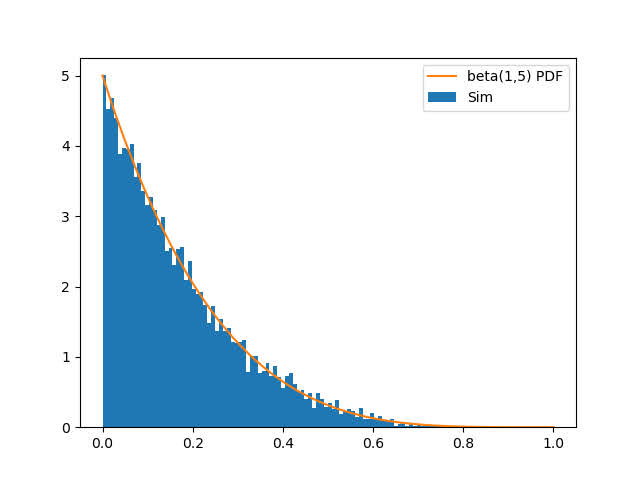For beta, the parameters a,b, the mean is a/b. To make the distribution more spread out, you have larger overall values of a/b (I don’t have the conversion to variance memorized offhand). But if you have real data, you can plop that into scipy to fit the parameters, here I fix the location and scale parameters.

``````# If you want to fit beta based on observed data
fitbeta = beta.fit(beta_prob,floc=0,fscale=1)``````

We can do similar for negative binomial, I often think of these in terms of regression dispersion parameters, and I have previously written about translating mean/dispersion to n/p notation:

``````# Ditto for negative binomial
mean_nb = 2
disp_nb = 4

def trans_np(mu,disp):
x = mu**2/(1 - mu + disp*mu)
p = x/(x + mu)
n = mu*p/(1-p)
return n,p

nb_n,nb_p = trans_np(mean_nb,disp_nb)
nb_sim = nbinom.rvs(nb_n,nb_p,size=(n,1))
nb_bars = pu(nb_sim)
plt.bar(nb_bars['Unique'],nb_bars['Percent'],label='Sim')

x = np.arange(0,nb_sim.max()+1)
plt.plot(x,nbinom.pmf(x,nb_n,nb_p)*100,linestyle='None',
marker='o',markerfacecolor='k',ms=7,
label=f'PMF Negative Binomial')
plt.legend()
plt.show()``````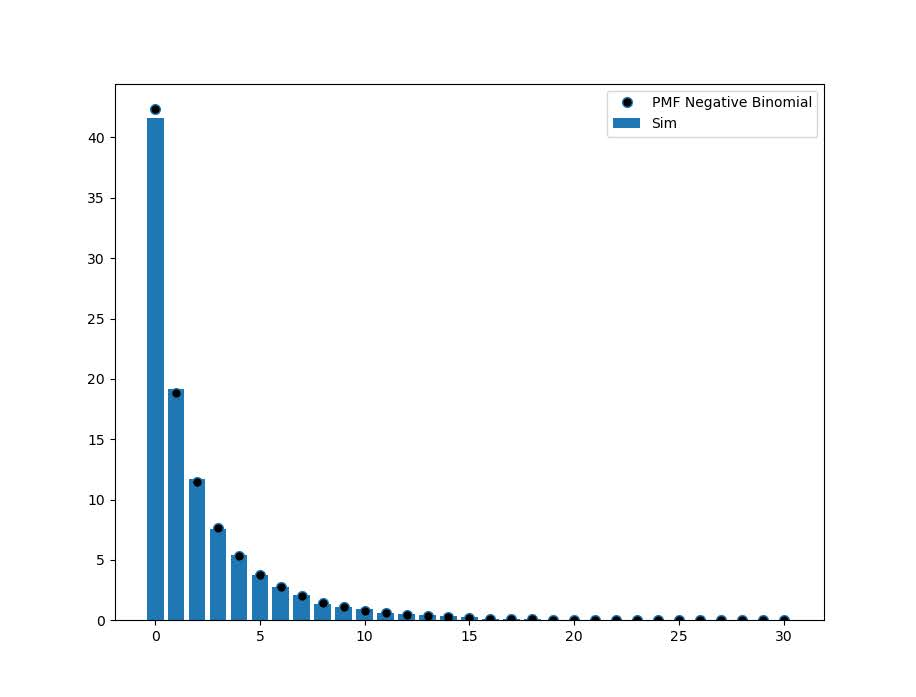I was working with some health data that only has MSA identifiers the other day. Not many people seem to know about the US Census’s FTP data site. Over the years they have had various terrible GUI’s to download data, but I almost always just go to the FTP site directly.

For geo data, check out https://www2.census.gov/geo/tiger/TIGER2019/ for example. Python for pandas/geopandas also has the nicety that you can point to a url (even a url of a zip file), and load in the data in memory. So to get the MSA areas was very simple:

``````# Example download MSA
import geopandas as gpd
from matplotlib import pyplot as plt

url_msa = r'https://www2.census.gov/geo/tiger/TIGER2019/CBSA/tl_2019_us_cbsa.zip'
msa.plot()
plt.show()``````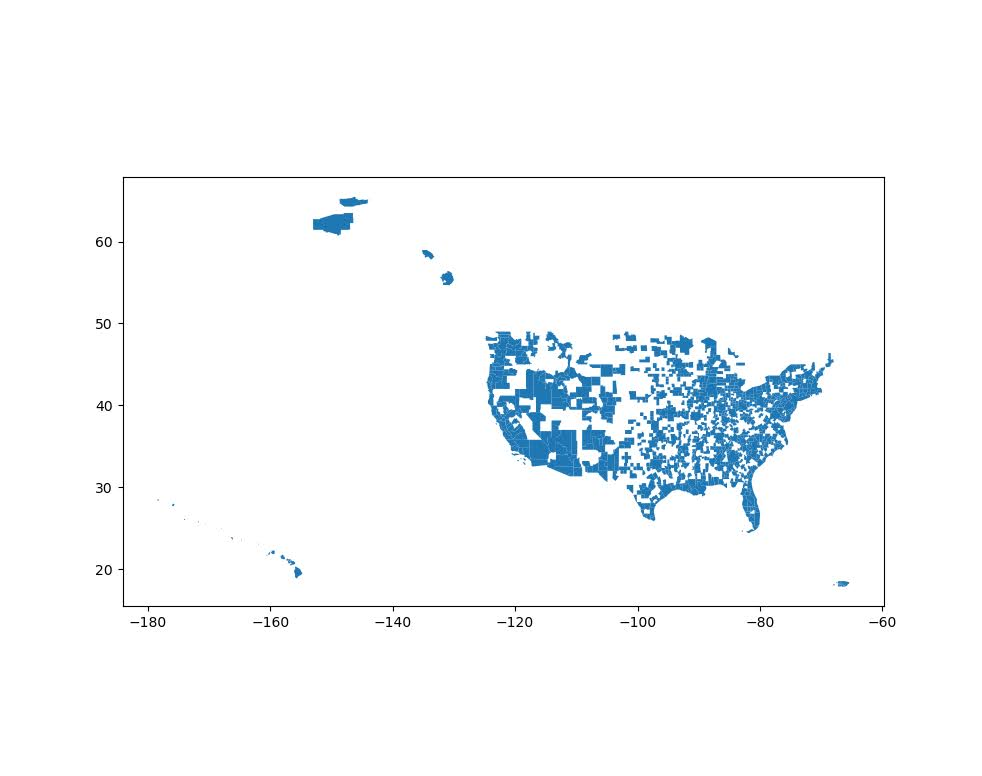Sometimes the census has files spread across multiple states. So here is an example of doing some simple scraping to get all of the census tracts in the US. You can combine the geopandas dataframes the same as pandas dataframes using `pd.concat`:

``````# Example scraping all of the zip urls on a page
from bs4 import BeautifulSoup
import pandas as pd
import re
import requests

def get_zip(url):
front_page = requests.get(url,verify=False)
soup = BeautifulSoup(front_page.content,'html.parser')
zf = soup.find_all("a",href=re.compile(r"zip"))
# Maybe should use href
zl = [os.path.join(url,i['href']) for i in zf]
return zl

base_url = r'https://www2.census.gov/geo/tiger/TIGER2019/TRACT/'
res = get_zip(base_url)

geo_tract = []
for surl in res:

geo_full = pd.concat(geo_tract)

# See State FIPS codes
# https://www.nrcs.usda.gov/wps/portal/nrcs/detail/?cid=nrcs143_013696

geo_full[geo_full['STATEFP'] == '01'].plot()
plt.show()``````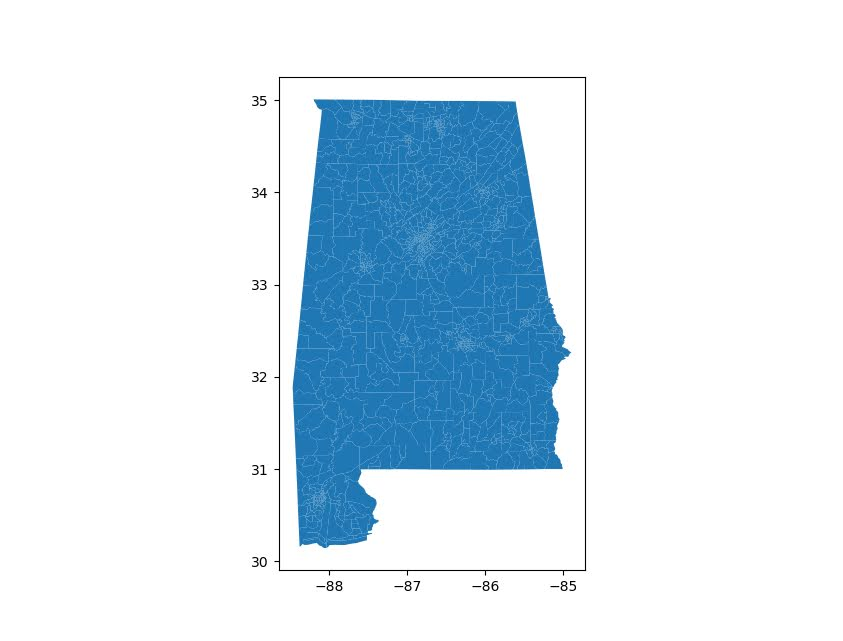Unfortunately for the census data tables, such as https://www2.census.gov/programs-surveys/acs/summary_file/2019/data/5_year_seq_by_state/Alabama/Tracts_Block_Groups_Only/, those zip files contain two files (an estimate file and a margin of error file), so you cannot just do `pd.read_csv(url)` for those tables. But for the shapefile zip files this appears to work just fine and dandy.

I am currently working on a project at work (but Gainwell has given me the thumbs up to open source it) to build tables to create the CDC’s Social Vulnerability Index, which I can build for multiple geographies in combo with the census data. So hopefully in the next few weeks will be able to share that work.

# Cointegration analysis of Ethereum and BitCoin

So a friend recently has heavily encouraged investment into Ethereum and NFTs. Part of the motivation of these cryptocurrencies is to be independent of fiat currency. So that lends itself to a hypothesis – are cryptocurrency prices and more typical securities independent? Or are we simply seeing similar trends in these different securities over time? This is a job for cointegration analysis. The python code is simple enough to follow along in a blog post.

So first I import the libraries I am using – it leverages the Yahoo finance API to download ticker data (here I analyze closing prices), and statsmodels to conduct the various analyses in python.

``````from datetime import datetime
import numpy as np
import pandas as pd
import yfinance as yf
import matplotlib.pyplot as plt

from statsmodels.tsa.api import VAR
from statsmodels.tsa.vector_ar import vecm``````

Now we can download the ticker data, here I will analyze BitCoin and Ethereum, along with Gold prices and the S&P 500 index fund.

``````# BTC-USD : Bitcoin
# ETH-USD : Ethereum
# ^GSPC ; S&P 500
# GC=F : Gold

end_date = datetime.now().strftime("%Y-%m-%d")
print(end_date) #running as of 2/9/2022

tick_str = 'BTC-USD ETH-USD ^GSPC GC=F'

Now for data prep – I am going to interpolate missing data (for when the market was closed). Then I only subset out Fridays at close to conduct a weekly analysis. Even weekly is too short for me to bother with rebalancing if I do decide to invest.

``````# Fill in missing data before sub-sampling to once a week
dat2 = dat.interpolate()

# Only Fridays close post 11/9/2017
dat2.reset_index(inplace=True)
after = pd.to_datetime('2017-11-09')
sel = (dat2.Date >= after) & (dat2.Date.dt.weekday == 4) #Friday
sdat = dat2.loc[sel,['Date','Close']]
sdat.columns = ['Date'] + ['BitCoin','Eth','S&P 500','Gold']
sdat.set_index('Date',inplace=True)``````

Now lets look at the overall trends by superimposing these four stocks on the same graph. Just min-max normalizing to range from 0 to 1.

``````# Time Series Graphs of Each
# Normalized to be 0/1
snorm = sdat.copy()
for v in sdat:
mi,ma = sdat[v].min(),sdat[v].max()
snorm[v] = (sdat[v] - mi)/(ma-mi)

# All four series on the same graph
snorm.plot(linewidth=2)
plt.show()``````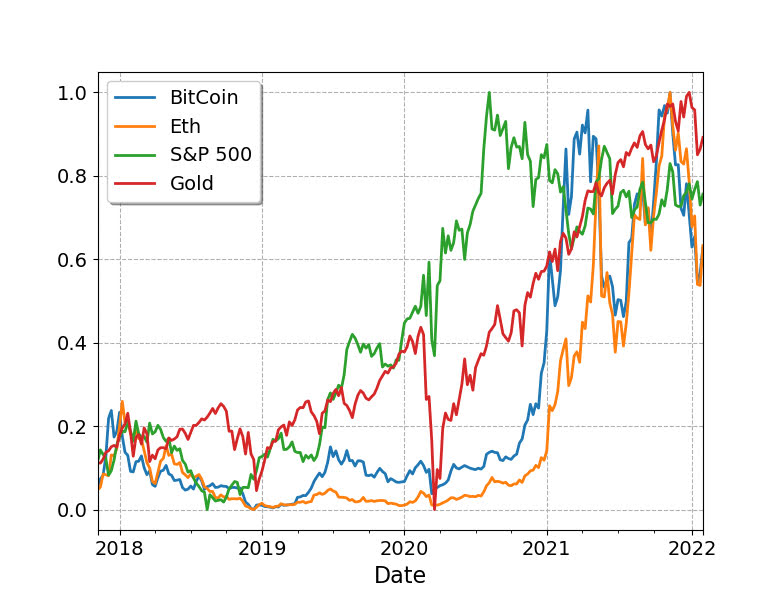So you can see these all appear to follow a similar upward trajectory after Covid hit in 2020, although crypto has way more volatility recently. If we subset out just the crypto’s, we can see how they trend with each other more easily.

``````s2 = sdat.copy()
s2['BitCoin/10'] = s2['BitCoin']/10
s2[['BitCoin/10','Eth']].plot(linewidth=2)
plt.show()``````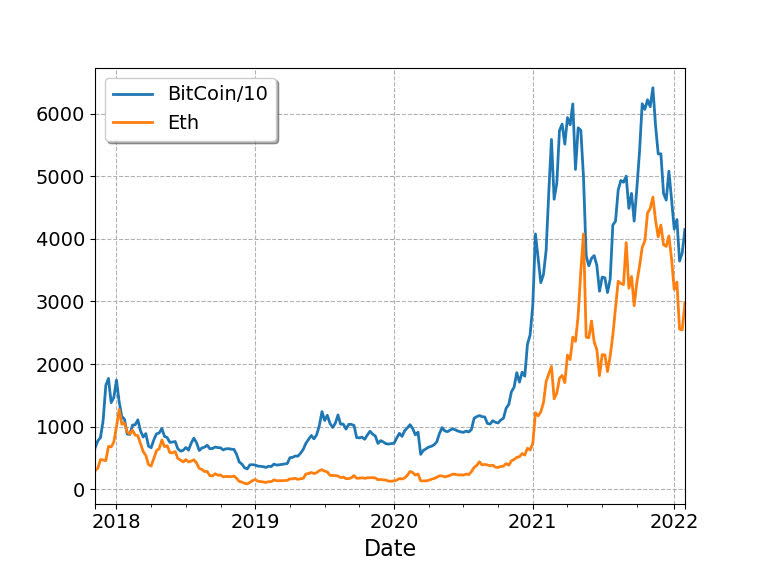So based on this, I would say that maybe Bitcoin is a leading indicator of Ethereum (increases in BitCoin precede increases in Eth with maybe just a week lag).

Typically with any time series analysis like this, we are concerned with whether the series are stationary. Just going off of my Ender’s Applied Econometric Time Series book, we typically look at the Adjusted Dickey-Fuller test for the levels:

``````# Integration analysis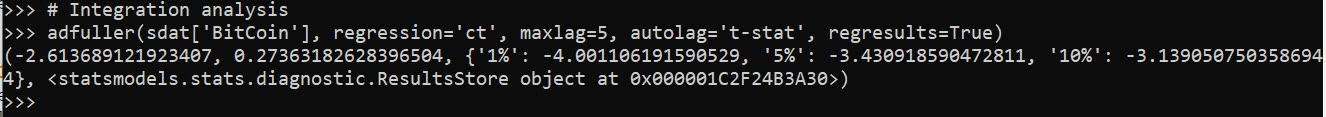And we can see this fails to reject the null, so we would conclude the series is integrated. Since we have a fairly large sample here (over 200 weeks), the test should be reasonably powered. If we then take the first differences and conduct the same test, we then reject the null of an integrated series (here for Bitcoin).

``````# Create differenced data
sdiff = sdat.diff().dropna()

# All appear 1st order integrated!So this reasonably suggests Bitcoin is an I(1) process. Doing the same for all of the other securities in this example you come to the same inference, all integrated of order 1 (which is very typical for stock data).

Using the differenced data, we can see the cross-correlations between different securities. In this example, it appears BitCoin/Ethereum just have a large 1 positive lag, and close to 0 after that.

``````# Only 1 lag positive in differenced data
pd.Series(ccf(sdiff['BitCoin'],sdiff['Eth'])[:10]).plot(kind='bar',grid=False)``````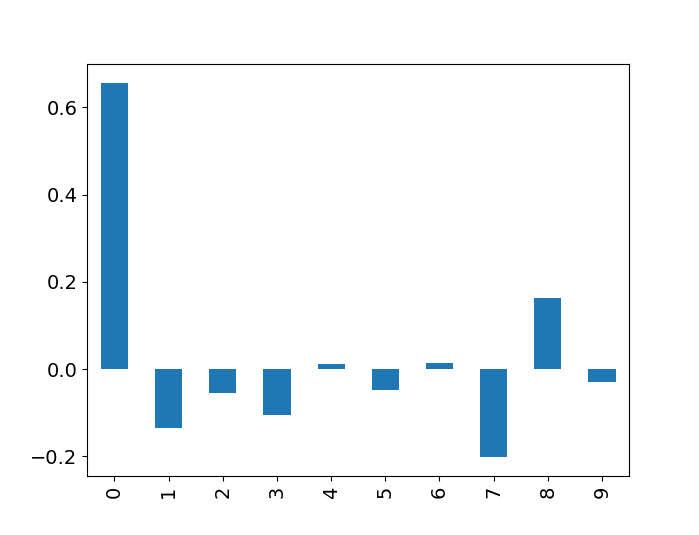So based on this, I subsequent only look at 1 lag in subsequent models. (Prior week impacts current week, since we are analyzing weekly data.)

So you need to be careful here – typically we want to avoid doing regression analysis of integrated time series, as that can lead to spurious correlations. But in the case a series is co-integrated, it is ok to conduct analysis on the levels. So here we do the analysis of the levels for each of the securities to assess our hypothesis. (Including temporal trends results in different coefficients, but similar overall inferences.)

``````mod = VAR(sdat)
res = mod.fit(1) #trend='ctt'
res.summary()``````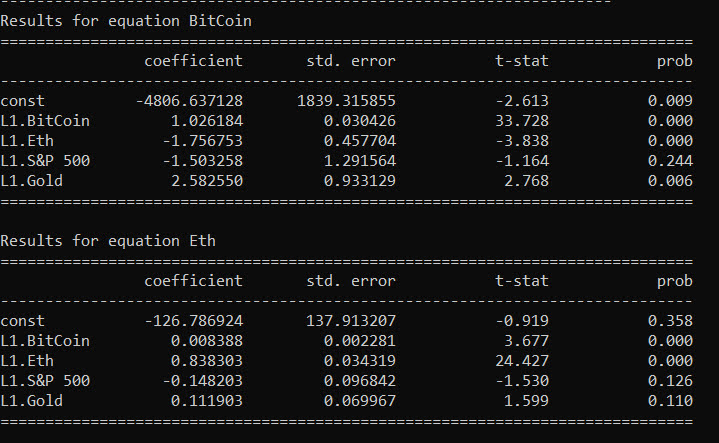So we can see here that contrary to the graphs, Ethereum has a negative relationship with BitCoin – when Ethereum goes up a dollar, the following week BitCoin goes down \$1.7. For BitCoin the relationships with S&P is negative (but weaker), and Gold it is positive.

``````# Ethereum causes BitCoin to go down
irf = res.irf(4)
irf.plot(orth=False,impulse='Eth',response='BitCoin')``````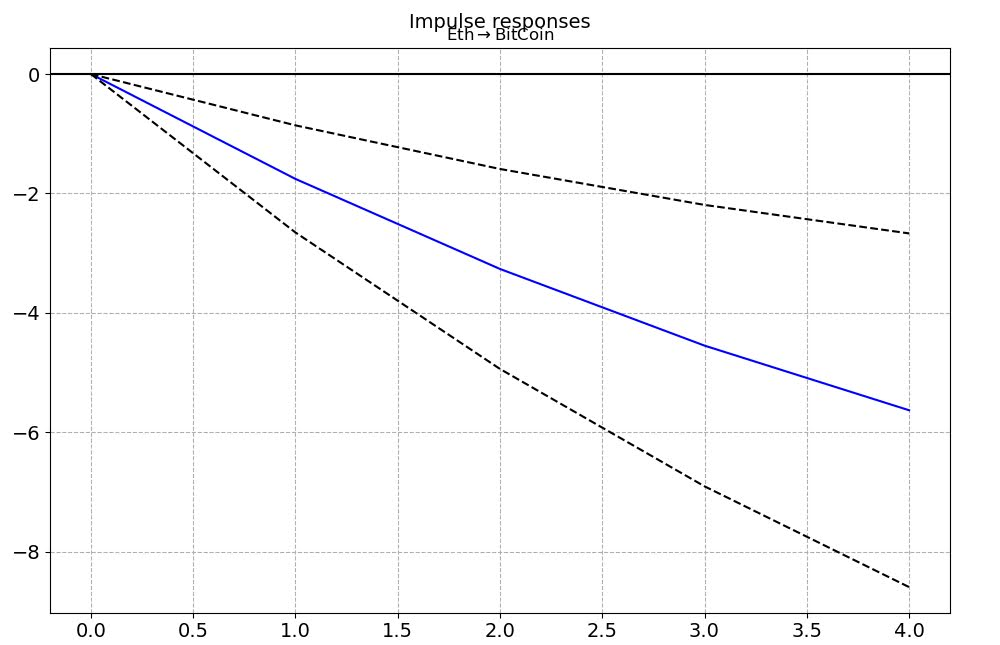For Ethereum the converse is not true though – BitCoin + increases Ethereum (although given that BitCoin is currently 10x the value of Eth the magnitude is smaller).

``````# Ethereum causes BitCoin to go down
irf = res.irf(4)
irf.plot(orth=False,impulse='Eth',response='BitCoin')``````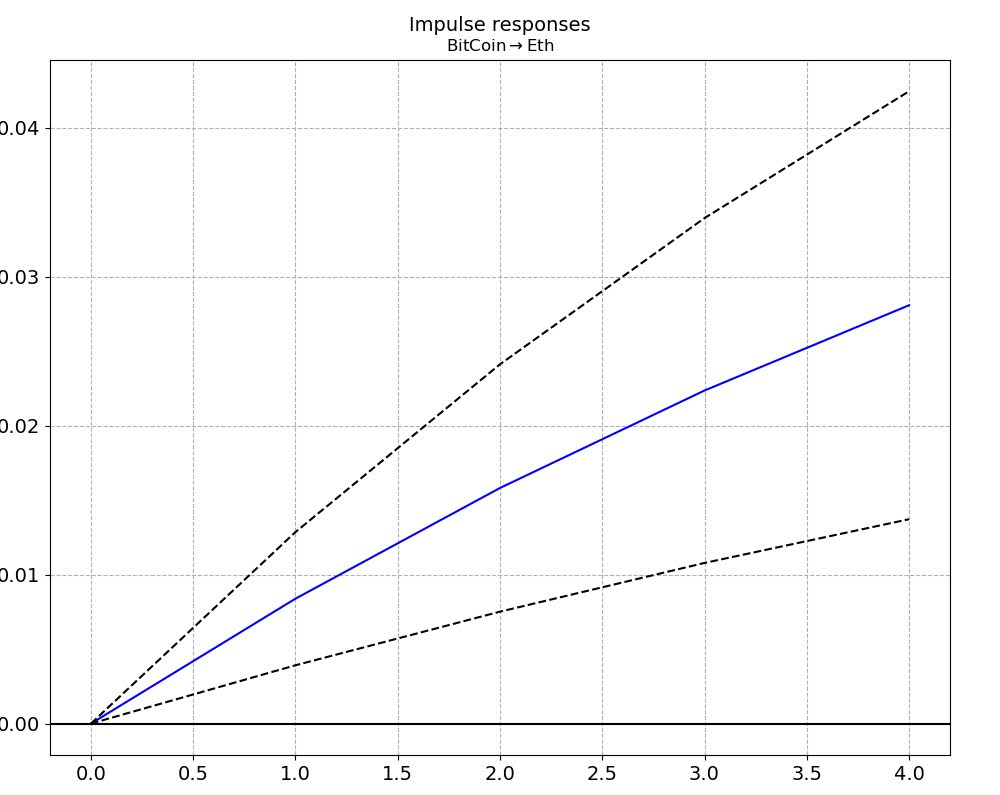There are more formal tests to look at Granger causality and cointegration with error correction models, but looking at the VAR of the levels I think is the easiest to Grok here.

Do not take this as investment advice, looking at the volatility of these securities makes me very hesistant to invest even a small sum.

``````# Granger causality test
gc = res.test_causality('Eth', 'BitCoin', kind='f').summary()
print(gc)

# Cointegration test
ecm = vecm.coint_johansen(sdat[['BitCoin','Eth']], 1, 1)
print(ecm.max_eig_stat)
print(ecm.max_eig_stat_crit_vals)

ecm = vecm.VECM(sdat[['BitCoin','Eth']],deterministic='co')
est = ecm.fit()

est.plot_forecast(4,n_last_obs=10)
plt.show()``````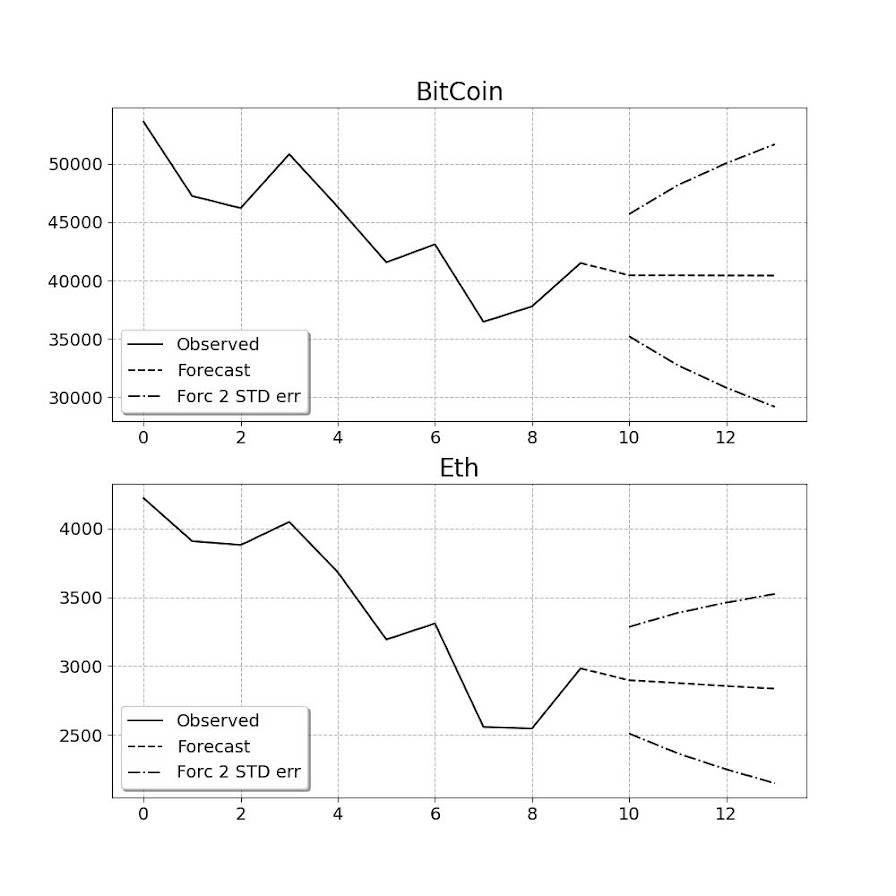Based on this analysis it might make sense to include BitCoin as a portfolio diversification relative to traditional stocks – if willing to assume quite a bit of risk. If you are a gambler it may make sense to do some type of pairs trading strategy between Eth/Bitcoin on a short term basis. (If I had some real magic low risk money making strategy I would not put it in a blog post!)

Gambling is fun (and it is fun to think damn if I invested in Eth in 2019 I would be up 10x) – but I don’t think I am going onto the crypto roller-coaster at the moment.

# Prediction Intervals for Random Forests

I previously knew about generating prediction intervals via random forests by calculating the quantiles over the forest. (See this prior python post of mine for getting the individual trees). A recent set of answers on StackExchange show a different approach – apparently the individual tree approach tends to be too conservative (coverage rates higher than you would expect). Those Cross Validated posts have R code, figured it would be good to illustrate in python code how to generate these prediction intervals using random forests.

So first what is a prediction interval? I imagine folks are more familiar with confidence intervals, say we have a regression equation `y = B1*x + e`, you often generate a confidence interval around `B1`. Imagine we use that equation to make a prediction though, `y_hat = B1*(x=10)`, here prediction intervals are errors around `y_hat`, the predicted value. They are actually easier to interpret than confidence intervals, you expect the prediction interval to cover the observations a set percentage of the time (whereas for confidence intervals you have to define some hypothetical population of multiple measures).

Prediction intervals are often of more interest for predictive modeling, say I am predicting future home sale value for flipping houses. I may want to generate prediction intervals that cover the value 90% of the time, and only base my decisions to buy based on the much lower value (if you are more risk averse). Imagine I give you the choice of buy a home valuated at `150k - 300k` after flipped vs a home valuated at `230k-250k`, the upside for the first is higher, but it is more risky.

In short, this approach to generate prediction intervals from random forests relies on out of bag error metrics (it is sort of like a for free hold out sample based on the bootstrapping approach random forest uses). And based on the residual distribution, one can generate forecast intervals (very similar to Duan’s smearing).

To illustrate, I will use a dataset of emergency room visits and time it took to see a MD/RN/PA, the NHAMCS data. I have code to follow along here, but I will walk through it in this post (that code has some nice functions for data definitions for the NHAMCS data).

At work I am working on a project related to unnecessary emergency room visits, and I actually went to the emergency room in December (for a Kidney stone). So I am interested here in generating prediction intervals for the typical time it takes to be served in an ER to see if my visit was normal or outlying.

# Example Python Code

First for some set up, I import the libraries I am using, and read in the emergency room use data:

``````import numpy as np
import pandas as pd
from nhanes_vardef import * #variable definitions
from sklearn.ensemble import RandomForestRegressor
from sklearn.model_selection import train_test_split

# Reading in fixed width data
# https://ftp.cdc.gov/pub/Health_Statistics/NCHS/Datasets/NHAMCS/
nh2019.columns = list(fw.keys())``````

Here I am only going to work with a small set of the potential variables. Much of the information wouldn’t make sense to use as predictors of time to first being seen (such as subsequent tests run). One thing I was curious about though was if I changed my pain scale estimate would I have been seen sooner!

``````# WAITTIME
# PAINSCALE [- missing]
# VDAYR [Day of Week]
# VMONTH [Month of Visit]
# ARRTIME [Arrival time of day]
# AGE [top coded at 95]
# SEX [1 female, 2 male]
# IMMEDR [triage]
#  9 = Blank
#  -8 = Unknown
#  0 = ‘No triage’ reported for this visit but ESA does conduct nursing triage
#  1 = Immediate
#  2 = Emergent
#  3 = Urgent
#  4 = Semi-urgent
#  5 = Nonurgent
#  7 = Visit occurred in ESA that does not conduct nursing triage

keep_vars = ['WAITTIME','PAINSCALE','VDAYR','VMONTH','ARRTIME',
'AGE','SEX','IMMEDR']
nh2019 = nh2019[keep_vars].copy()``````

Many of the variables encode negative values as missing data, so here I throw out visits with a missing waittime. I am lazy though and the rest I keep as is, with enough data random forests should sort out all the non-linear effects no matter how you encode the data. I then create a test split to evaluate the coverage of my prediction intervals out of sample for 2k test samples (over 13k training samples).

``````# Only keep wait times that are positive
mw = nh2019['WAITTIME'] >= 0
print(nh2019.shape - mw.sum()) #total number missing
nh2019 = nh2019[mw].copy()

# Test hold out sample to show
# If coverage is correct
train, test = train_test_split(nh2019, test_size=2000, random_state=10)
x = keep_vars[1:]
y = keep_vars``````

Now we can fit our random forest model, telling python to keep the out of bag estimates.

``````# Fitting the model on training data
regr = RandomForestRegressor(n_estimators=1000,max_depth=7,
random_state=10,oob_score=True,min_samples_leaf=50)
regr.fit(train[x], train[y])``````

Now we can use these out of bag estimates to generate error intervals around our predictions based on the test oob error distribution. Here I generate 50% prediction intervals.

``````# Generating the error distribution
resid = train[y] - regr.oob_prediction_
# 50% interval
lowq = resid.quantile(0.25)
higq = resid.quantile(0.75)
print((lowq,higq))
# negative much larger
# so tends to overpredict time``````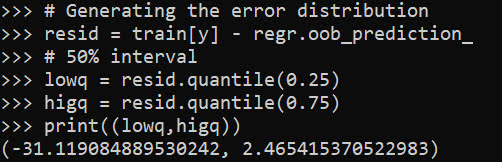Even 50% here are quite wide (which could be a function of both the data has a wide variance as well as the model is not very good). But we can test whether our prediction intervals are working correctly by seeing the coverage on the out of sample test data:

``````# Generating predictions on out of sample data
test_y = regr.predict(test[x])
lowt = (test_y + lowq).clip(0) #cant have negative numbers
higt = (test_y + higq)

cover = (test[y] >= lowt) & (test[y] <= higt)
print(cover.mean())``````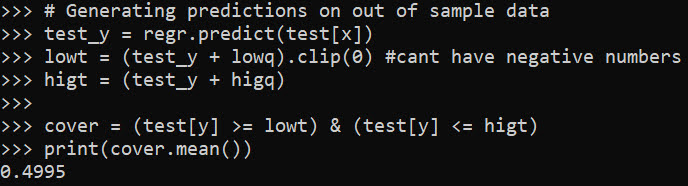Pretty much spot on. So lets see what the model predicts my referent 50% prediction interval would be (I code myself a 2 on the `IMMEDR` scale, as I was billed a CPT code 99284, which those should line up pretty well I would think):

``````# Seeing what my referent time would be
myt = np.array([[6,4,12,930,36,2,6]])
mp = regr.predict(myt)
print(mp)
print( (mp+lowq).clip(0), (mp+higq) )``````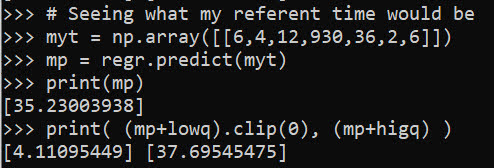So a predicted mean of 35 minutes, and a prediction interval of 4 to 38 minutes. (These intervals based on the residual quantiles are basically non-parametric, and don’t have any strong assumptions about the distribution of the underlying data.)

To first see the triage nurse it probably took me around 30 minutes, but to actually be treated it was several hours long. (I don’t think you can do that breakdown in this dataset though.)

We can do wider intervals, here is a screenshot for 80% intervals: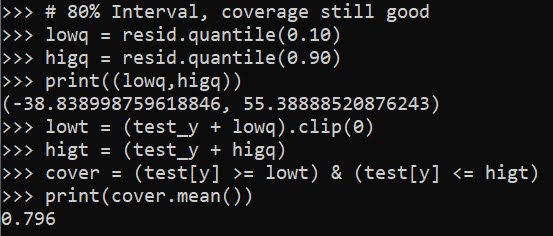You can see that they are quite wide, so probably not very effective in identifying outlying cases. It is possible to make them thinner with a better model, but it may just be the variance is quite wide. For folks monitoring time it takes for things (whether time to respond to calls for service for police, or here be served in the ER), it probably makes sense to build models focusing on quantiles, e.g. look at median time served instead of mean.

# Optimal and Fair Spatial Siting

A bit of a belated MLK day post. Much of the popular news on predictive or machine learning algorithms has a negative connotation, often that they are racially biased. I tend to think about algorithms though in almost the exact opposite way – we can adjust them to suit our objectives. We just need to articulate what exactly we mean by fair. This goes for predictive policing (Circo & Wheeler, 2021; Liberatore et al., 2021; Mohler et al., 2018; Wheeler, 2020) as much as it does for any application.

I have been reading a bit about spatial fairness in siting health resources recently, one example is the Urban Institutes Equity Data tool. For this tool, you put in where your resources are currently located, and it tells you whether those locations are located in areas that have demographic breakdowns like the overall city. So this uses the container approach (not distance to the resources), which distance traveled to resources is probably a more typical way to evaluate fair spatial access to resources (Hassler & Ceccato, 2021; Koschinsky et al., 2021).

Here what I am going to show is instead of ex-ante saying whether the siting of resources is fair, I construct an integer linear program to site resources in a way we define to be fair. So imagine that we are siting 3 different locations to do rapid Covid testing around a city. Well, we do the typical optimization and minimize the distance traveled for everyone in the city on average to those 3 locations – on average 2 miles. But then we see that white people on average travel 1.9 miles, and minorities travel 2.2 miles. So it that does not seem so fair does it.

I created an integer linear program to take this difference into account, so instead of minimizing average distance, it minimizes:

``White_distance + Minority_distance + |White_distance - Minority_distance|``

So in our example above, if we had a solution that was white travel 2.1 and minority 2.1, this would be a lower objective value than (4.2), than the original minimize overall travel (1.9 + 2.2 + 0.3 = 4.4). So this gives each minority groups equal weight, as well as penalizes if one group (either whites or minorities) has much larger differences.

I am not going to go into all the details. I have python code that has the functions (it is very similar to my P-median model, Wheeler, 2018). The codes shows an example of siting 5 locations in Dallas (and uses census block group centroids for the demographic data). Here is a map of the results (it has points outside of the city, since block groups don’t perfectly line up with the city boundaries).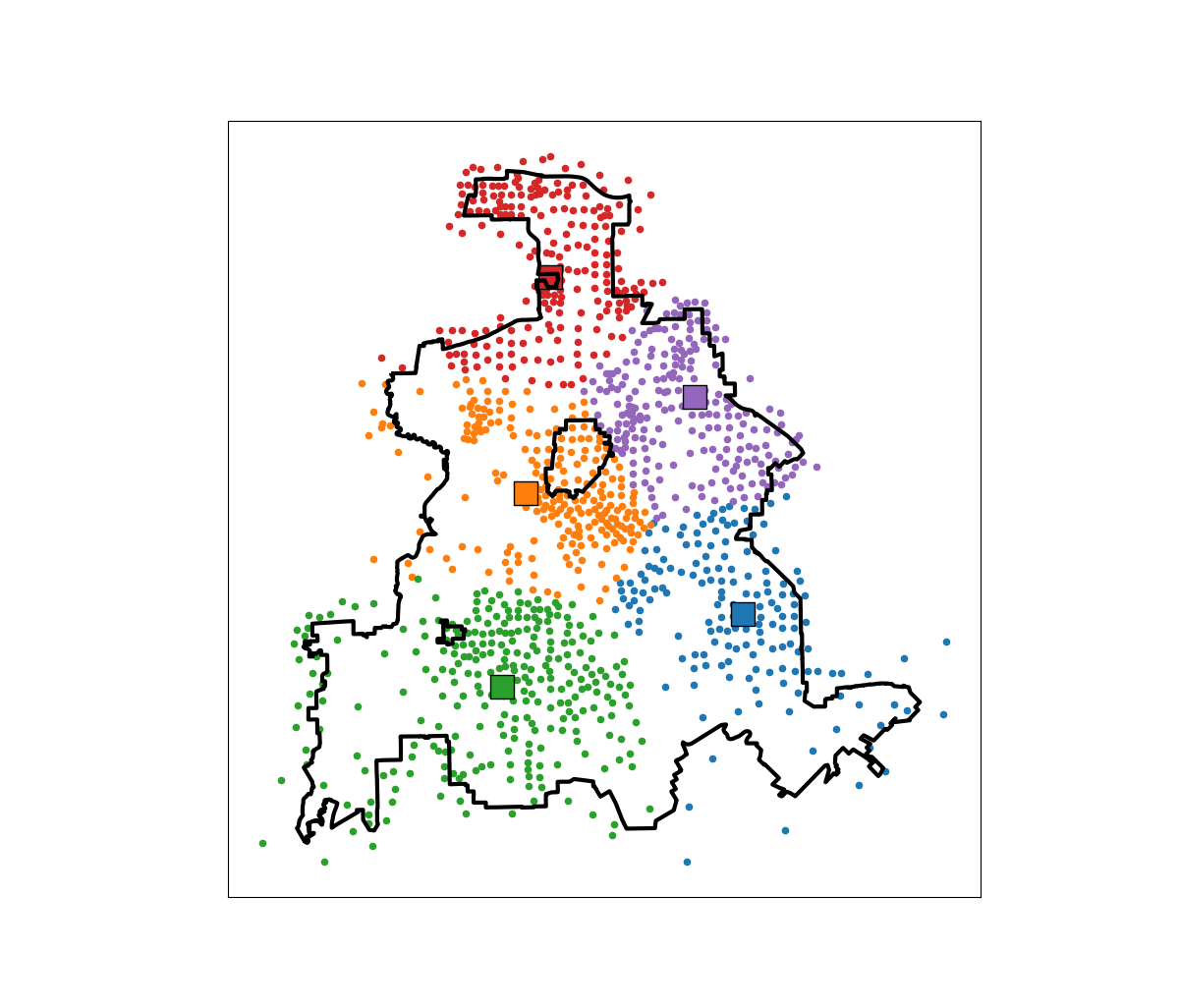In this example, if we choose 5 locations in the city to minimize the overall distance, the average travel is just shy of 3.5 miles. The average travel for white people (not including Hispanics) is 3.25 miles, and for minorities is 3.6 miles. When I use my fair algorithm, the white average distance is 3.5 miles, and the minority average distance is 3.6 miles (minority on average travels under 200 more feet on average than white).

So this is ultimately a trade off – it ends up pushing up the average distance a white person will travel, and only slightly pushes down the minority travel, to balance the overall distances between the two groups. It is often the case though that one can be somewhat more fair, but in only results in slight trade-offs though in the overall objective function (Rodolfa et al., 2021). So that trade off is probably worth it here.